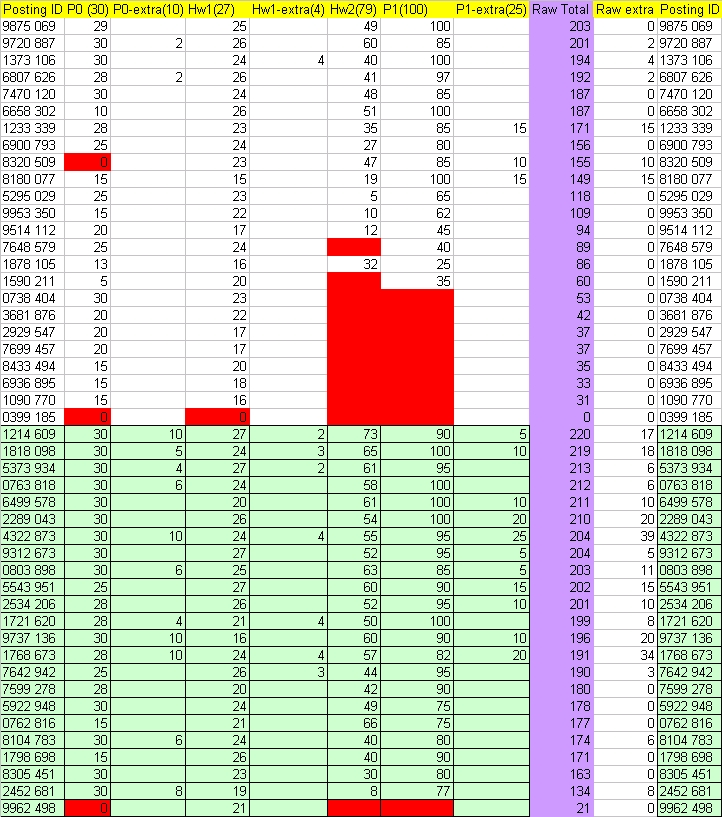# Current raw totals

Folks:
Here are the current raw totals (Project 0 and 1; Homeworks 1 and 2). The UGs (white) and Grads (Green) are
sorted into two groups. Each group is sorted in the descending order of Raw Total (purple column). Raw extra marks are kept
separate. Please note that these are RAW (unweighted) totals. The two homeworks and the project 0 and 1 together
won't probably count for more than about 25% of your overall grade (exact percentage depends on how many homeworks and
projects get given).